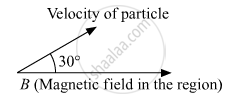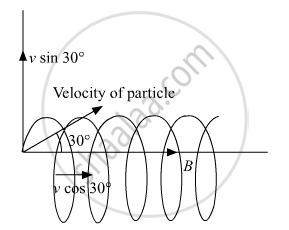# A Charged Particle Q is Moving in the Presence of a Magnetic Field B Which is Inclined to an Angle 30° with the Direction of the Motion of the Particle. - Physics

A charged particle q is moving in the presence of a magnetic field B which is inclined to an angle 30° with the direction of the motion of the particle. Draw the trajectory followed by the particle in the presence of the field and explain how the particle describes this path.

#### SolutionLorentz force will be exerted on the particle because of the Sine component of particle velocity thereby making the particle move in a helical path.

Time period = ("Total distance")/("speed") =  (2"πR")/("v"_"y") (2"πR")/("v" sin 30°) = (4"πR")/("v")

Pitch = speed along x-axis × time period

= "v"_x xx (2"πR")/("v"_"y")

= "v"_x xx (2"πR")/("v"sin30°) = "v"_x xx (2"πR" xx 2)/("v")

= "v" cos 30° xx (4"πR")/("v") = sqrt(3)/(2) xx 4"πR" = 2sqrt(3)"πR"

R = (mv sin 30°)/(q"B")

Pitch = (2sqrt(3)"πmv" sin 30°)/(q"B")

= (2sqrt(3)"πmv")/(q"B" xx2)

= (sqrt(3)"πmv")/(q"B")

The vertical component of velocity will make the charged particle to move in a circular path whereas the horizontal component of velocity will provide  Pitch = (sqrt(3)"πmv")/(q"B") Hence the motion of the particle will be helical with the Pitch= (sqrt(3)"πmv")/(q"B").

Concept: Alpha-particle Scattering and Rutherford’S Nuclear Model of Atom
Is there an error in this question or solution?